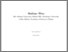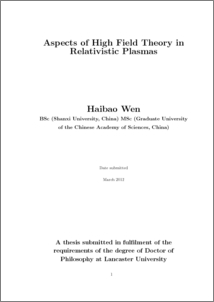# Aspects of high field theory in relativistic plasmas

Wen, Haibao (2012) Aspects of high field theory in relativistic plasmas. PhD thesis, UNSPECIFIED.Preview
PDF (2012WenPhD)
thesis_Wen.pdf - Published Version

## Abstract

This thesis is concerned with plasmas and high field physics. We investigate the oscillations of relativistic plasmas using a kinetic description (Chapter II), a macroscopic fluid moment description (Chapter III), a quantum description (Chapter IV as a brief exploration) and Born-Infeld electrodynamics (Chapter V). Using a kinetic description, we examine the non-linear electrostatic oscillations of waterbag-distributed plasmas and obtain the maximum electric field Emax (Chapter II). Using a macroscopic fluid moment description with the closure of the Equations Of State (EOSs), we obtain the maximum electric field Emax of electrostatic oscillations for various waterbag-distributed electron fluids, which may imply the advantages of some fluids with particular EOSs in the aspect of particle acceleration. Furthermore, we find that fluids with a more general class of EOSs may have the same advantages (Chapter III). A brief numerical calculation of an ODE system originating from the Maxwell equations and a Madelung decomposition of the Klein-Gorden equation with a U(1) field shows that electrostatic oscillations decay in a Klein-Gorden plasma due to quantum effects (Chapter IV). With calculations using the Born-Infeld equations and the Lorentz equation, we investigate the electrostatic and electromagnetic oscillations in cold plasmas in Born-Infeld electrodynamics (Chapter V). For the electrostatic oscillations we find that the electric field of Born-Infeld electrodynamics behaves differently from that of Maxwell electrodynamics. However, Born-Infeld electrodynamics gives the same prediction as Maxwell electrodynamics for the maximum energy that a test electron may obtain in an electrostatic wave (Section VA). For electromagnetic waves, the dispersion relation and the cutoff frequencies of the “R”, “L” and “X” modes of electromagnetic waves in Born-Infeld cold plasma are deduced to be different from those in Maxwell cold plasma. The cutoff frequencies (when the index of refraction n → 0) are also obtained, showing the advantage of “O” mode waves for the acceleration of particles (Section VB).

Item Type:
Thesis (PhD)
Departments:
ID Code:
76598
Deposited By:
Deposited On:
10 Nov 2015 17:18
Refereed?:
No
Published?:
Published